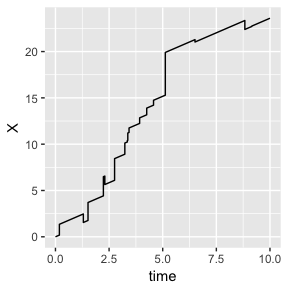# A Very Short Introduction to ruin

#### 2018-07-30

In the framework of risk theory, an insurance company is modeled as a stochastic process of its capital. Once the capital of such company falls below zero, the company is considered as bankrupt or ruined. Therefore, one of the key problems of the risk theory is to assess and estimate the probability of ruin. For many classical cases, the ruin probability can be derived explicitly, as a function of the model parameters. Usually, however, for more complex models the solution can be found only numerically, or even using Monte-Carlo simulation methods.

The package ruin is the first attempt to formally define various risk processes in R environment by using S4 object-oriented methodology. Each model is supposed to have its own simulator that allows estimating the ruin probability. The current version includes only the simplest models, for most of which the ruin probabilities are known.

This vignette proposes a toy example of how to use the package. We consider a Cramér-Lundberg’s extension that includes capital injections, which is defined as follows:

$X(t) = u + ct + \sum_{i=1}^{N^{(+)}(t)} Y^{(+)}_i - \sum_{k=1}^{N^{(-)}(t)} Y^{(-)}_k,$

where:

• $$u$$: the initial capital ($$u \geq 0$$),
• $$c$$: the premium rate ($$c \geq 0$$),
• $$N^{(+)}(t)$$ is the Poisson process of capital injections with the intensity $$\lambda^{(+)} > 0$$,
• $$Y^{(+)}_i$$ are iid capital injections’ sizes,
• $$N^{(-)}(t)$$ is the Poisson process of claims with the intensity $$\lambda^{(-)} > 0$$,
• $$Y^{(-)}_k$$ are iid claims’ sizes.

This model is implemented as the S4 class CramerLundbergCapitalInjections. In order to create an object of this class, the constructor of the same name should be used:

library(ruin)
set.seed(1991)

model <- CramerLundbergCapitalInjections(
initial_capital = 0,
claim_poisson_arrival_rate = 1,
claim_size_generator = rexp,
claim_size_parameters = list(rate = 1),
capital_injection_poisson_rate = 1,
capital_injection_size_generator = rexp,
capital_injection_size_parameters = list(rate = 1)
)

The arguments’ names of the constructor are self-explanatory: initial_capital defines the initial capital $$u$$, etc.

A generic function simulate_path(), as can be guessed from its name, simulates one path of the given model:

one_path <- simulate_path(model = model, max_time_horizon = 10)

The function is dispatched for all implemented models and returns an object of the corresponding class Path* (e.g., passing CramerLundberg as a model will yield an object of PathCramerLundberg). This object contains various information about the realization: the underlying model, path itself, whether the path was ruined, the random seed, etc:

one_path <- simulate_path(model = model, max_time_horizon = 10)

#>           time         X
#> [1,] 0.0000000 0.0000000
#> [2,] 0.1830452 0.1830452
#> [3,] 0.1830452 1.3492343
#> [4,] 1.2995801 2.4657691
#> [5,] 1.2995801 1.5462556
#> [6,] 1.5177418 1.7644173

Further, plot_path() function can be used for visualizing the simulated path:

plot_path(one_path)However, the main function of the package is ruin_probability(), which returns a Monte-Carlo estimate of ruin probability for the finite time. Under the hood, the function simulates a vast number of paths (possibly using a parallel computing) and divides the number of ruined processes over the total number of simulations:

ruin_probability(model = model, time_horizon = 10, parallel = FALSE)
#> \$ruin_probability
#> lower_bound    estimate upper_bound
#>   0.5677178   0.5774000   0.5870822